# Tetris Tiling Algorithm

Python algorithm development and optimisation
Module
Computing
Competition
7th fastest in a class of 65
The challenge was to make a Python algorithm to find the most accurate solution to a target pattern of tetrominoes as quickly as possible. I used a multiway tree and recursion to tile the target pattern quicklz and accurately.
Development Process
To begin with, I’ve drawn out the target grid and tried to fill it with tetrominoes manually, on paper. By doing so I identified the possible approaches to solving the problem. I then identified the steps I took to achieve the solution and translated them first into pseudo code and then into Python code.

The code was then tested on target matrices of varying sizes from 10x10 up to 1000x1000 and densities from 0.2 to 0.8. Iterations to previously written code were highlighted alongside with dates of the edit for future reference and easier debugging.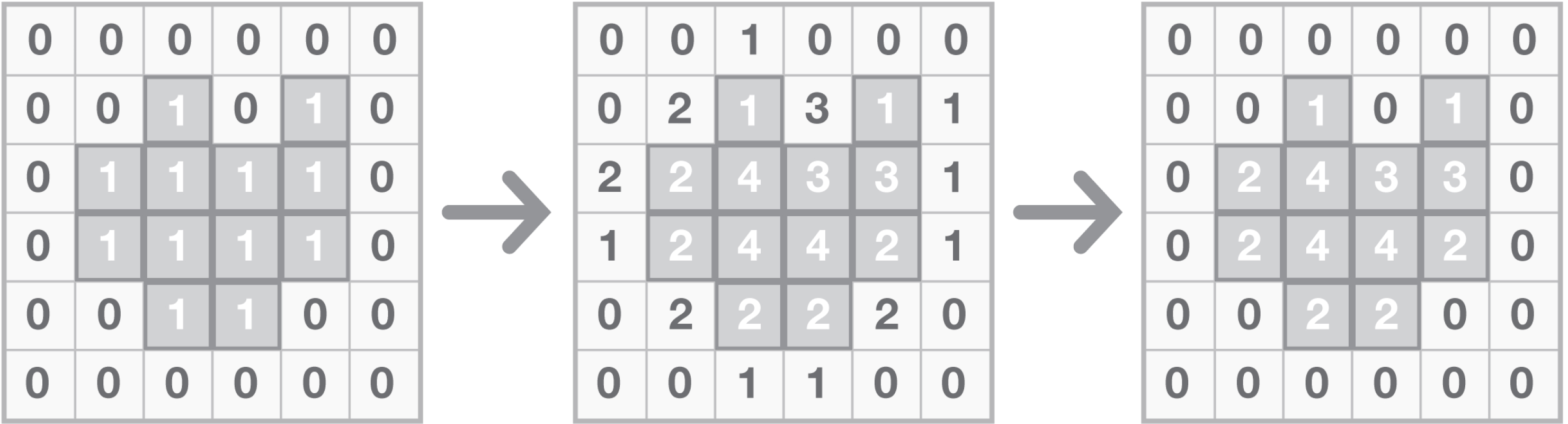Weighting
Firstly, a new matrix is created with the number of neighbours of a tile as the value of that tile. The code is scanned row by row and each neighbours of each coordinate are checked. The neighbour value is then added to a variable. After this, the coordinates with zeros in the target are reset to zeros in the weighted matrix. This is done to prevent placement of shapes onto empty tiles. The weighted matrix is then padded with three rows of zeroes to prevent Index errors when placing shapes and calculating weighting.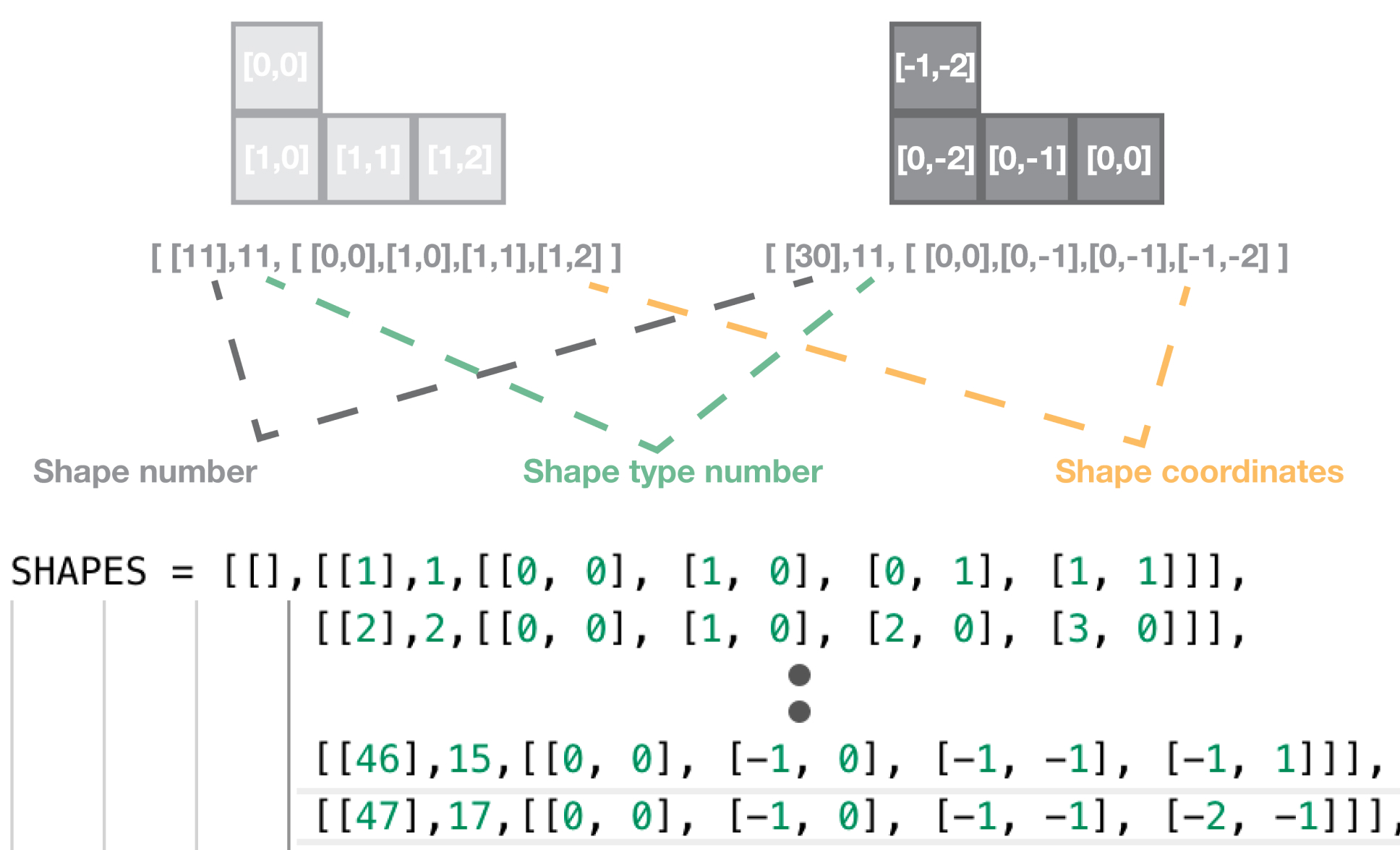Tetriling Shape Representation In Code
The shapes are defined as a list with a shape number, shape type number and a list of its co- ordinates. There is 19 shape types and 47 shape numbers. This is because one shapes is defined from more origins, making it possible to be identified when any tile of the shape is checked first.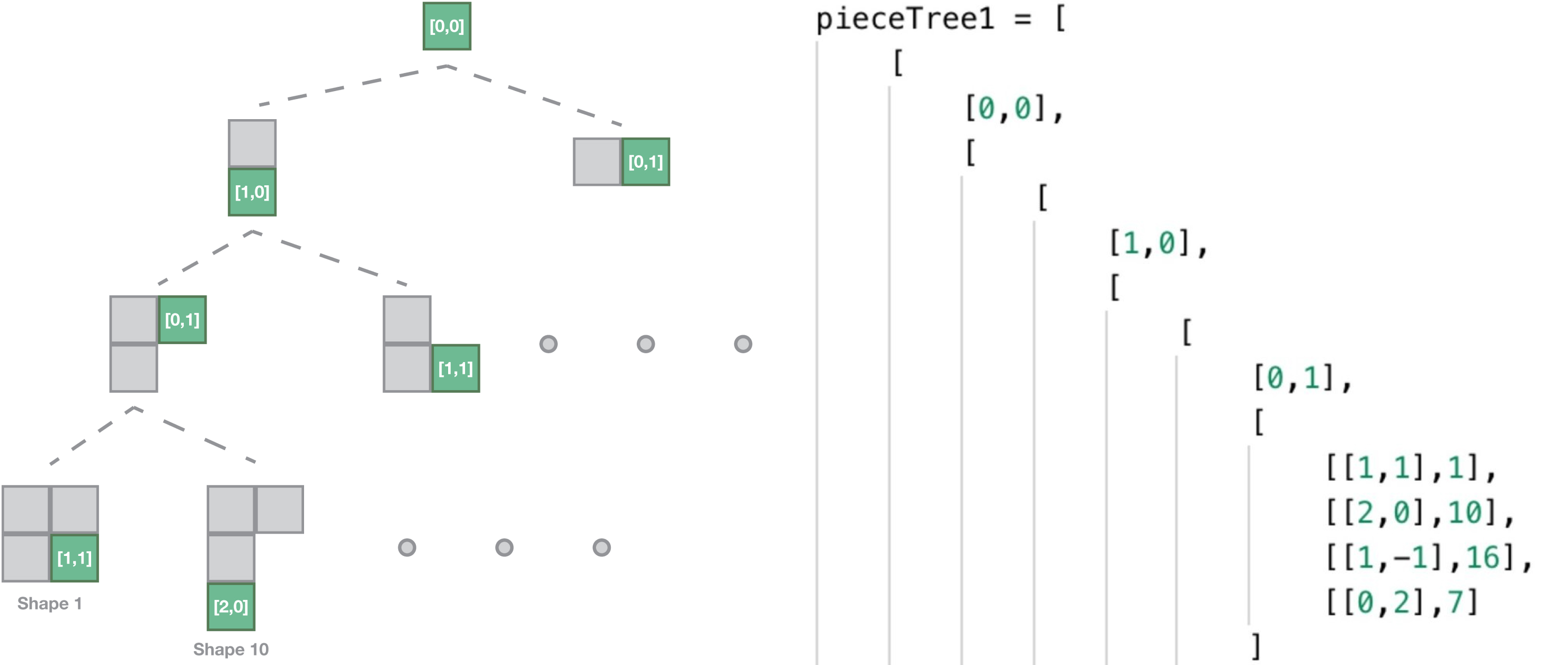Problem Representation In The Form Of a Tree
The shapes are defined in a multiway tree to reduce the number of checks needed to find whether a shape fits into a position. Implementing a tree reduced the number of checks from 19 when going through all the shapes one by one to a maximum of 6 checks. The root node is 0,0 - the origin. It has 4 children, [0,1], [1,0], [0,-1] [-1,0] so that shapes can be checked in all directions from the origin.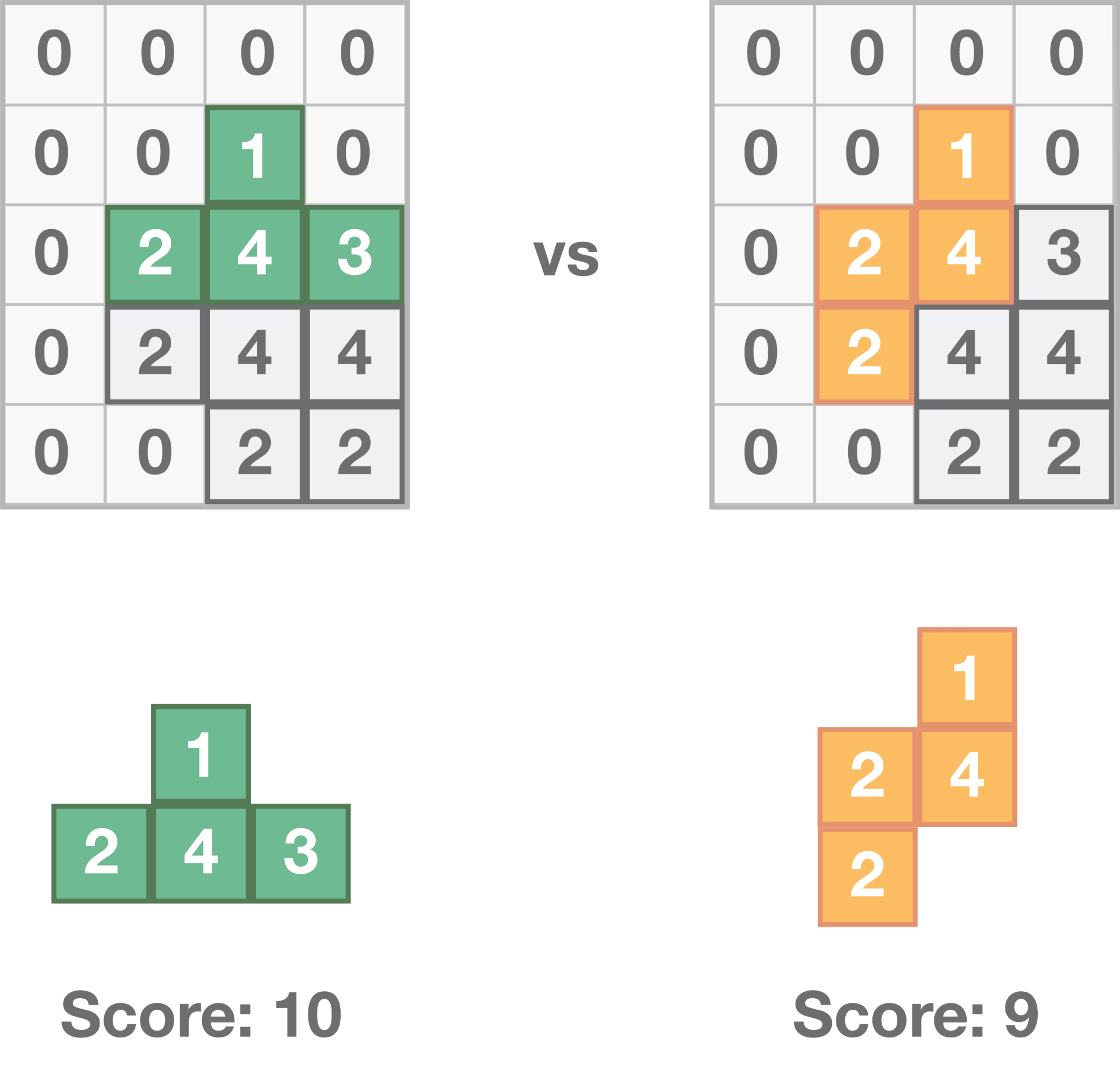Placing Shapes
Weighting of each of the shapes is calculated - by summing the weightings of the tile values on which it would be placed. The weightings are then sorted from lowest to highest and stored in a two dimensional list.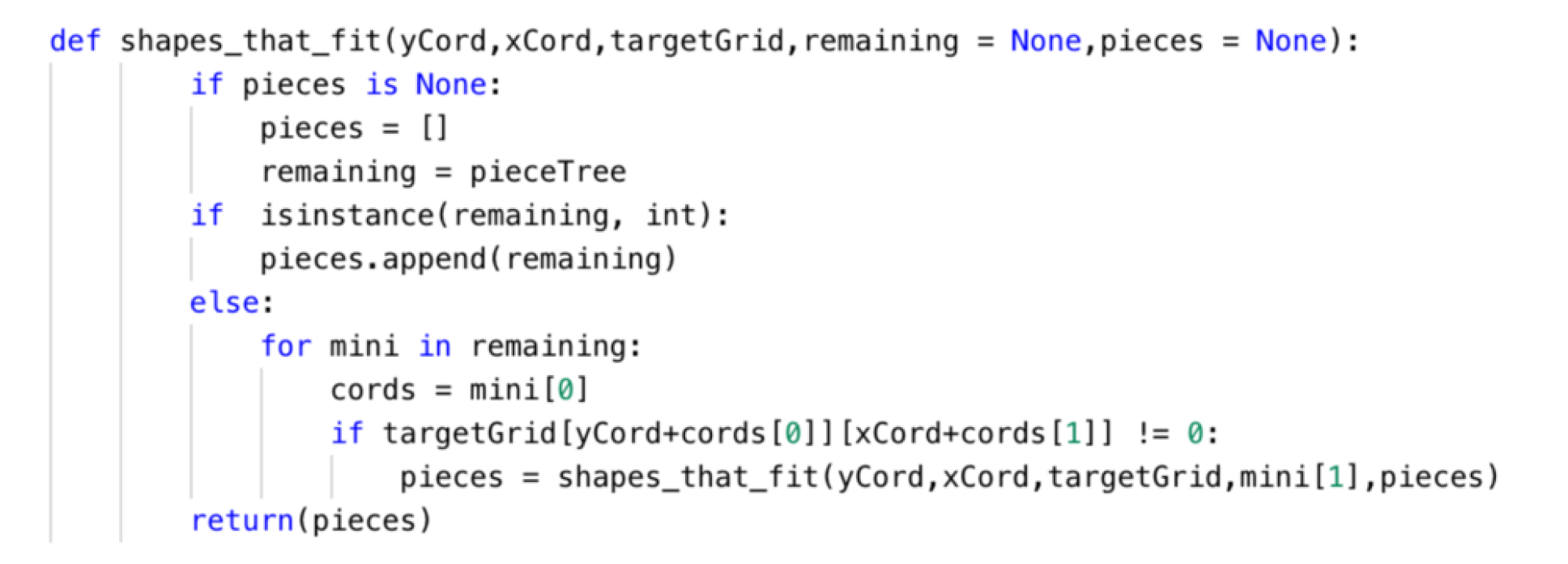Depth First Tree Traversal Using Recursion
The algorithm starts by scanning the weighted and padded matrix for tiles with weighting of one. This essentially allows the algorithm start from the edge as a tile with a weighting of one has only one neighbour and is protruding from its connected component. When a tile with weighting of one is found, recursion and depth first search is used to traverse down the tree and output a list of shape numbers of shapes that would fit if placed from the origin that is being currently checked. If there is no tile placed at a coordinate defined in a node of the tree, the whole subtree of that node is removed. Weighting of each of the shapes is calculated - by summing the weightings of the tile values on which it would be placed. The weightings are then sorted from lowest to highest and stored in a two dimensional list.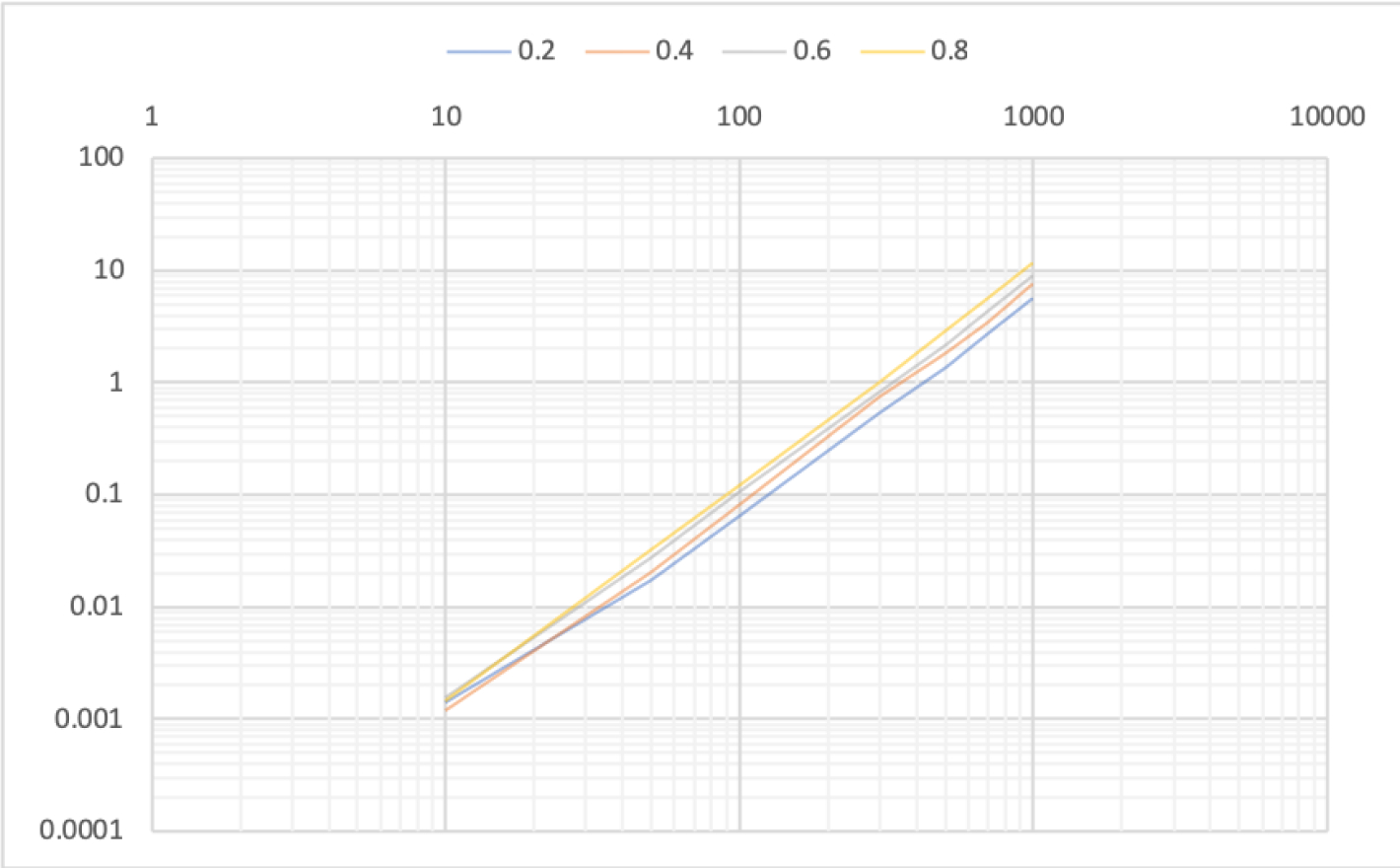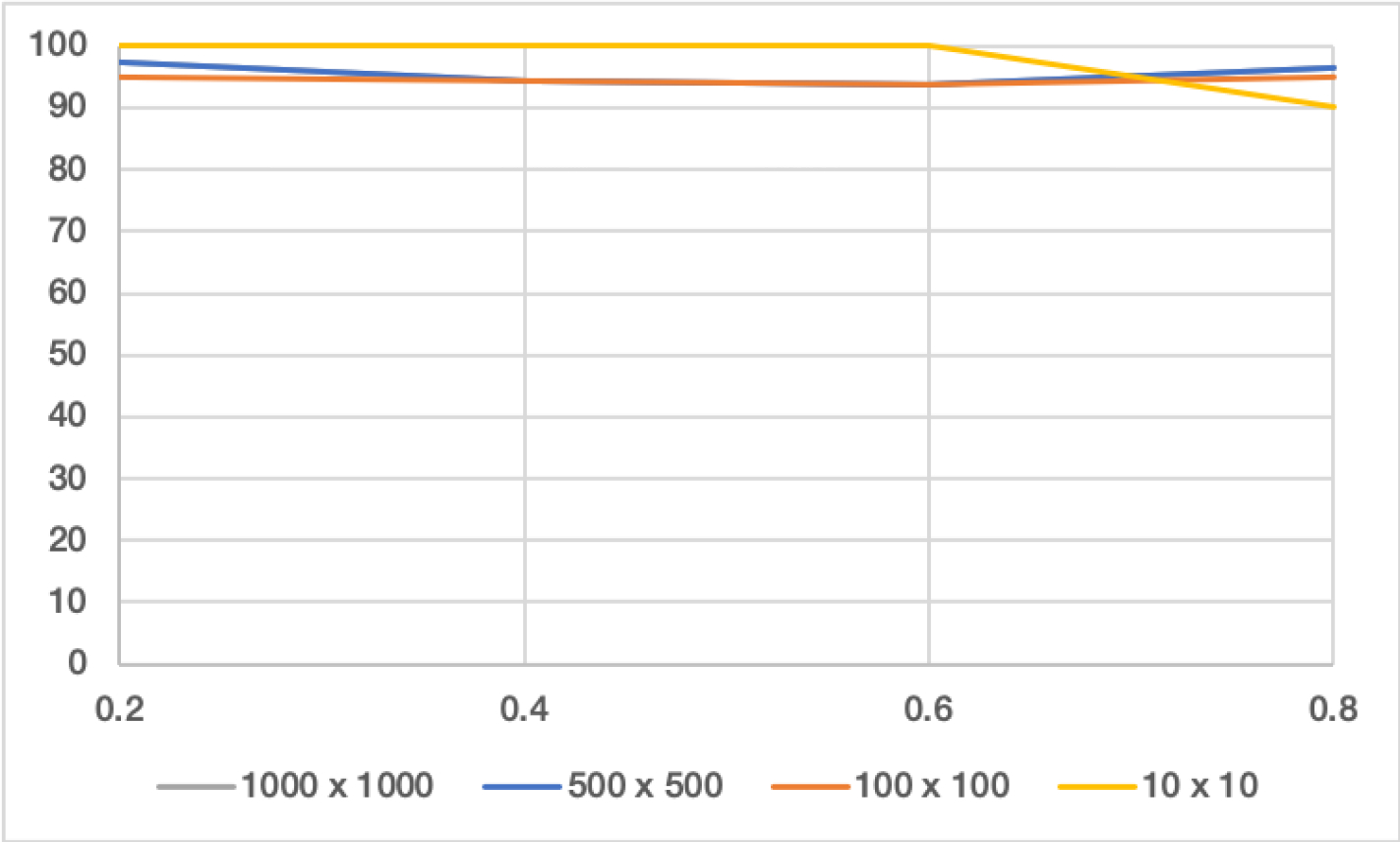Runtimes and Accuracy
All of the functions used in the algorithm are linear with respect to the area of the target grid. The overall running time of the algorithm is estimated to be O(n), where n is the area of the target matrix.

The accuracy of the algorithm ranges from 93% for smaller targets to 98% for larger sized targets.